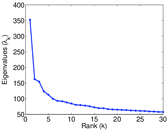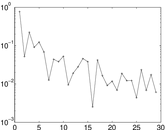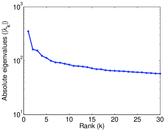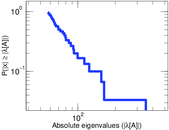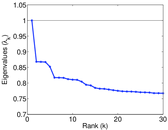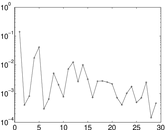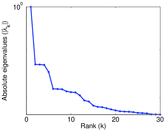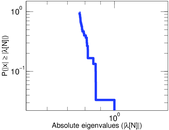# TV Tropes

This is the bipartite network of TV Tropes (tvtropes.org), characterising artistic works by their tropes. Each edge connects a work (such as a movie, a novel, etc.) to one trope (stylistic convention or device). The network was extracted by the DBTropes project (dbtropes.org).

 Code `DBT` Internal name `dbtropes-feature` Name TV Tropes Data source http://dbtropes.org/ AvailabilityDataset is available for download Consistency checkDataset passed all tests Category Feature network Node meaning Work, trope Edge meaning HasFeature Network formatBipartite, undirected Edge typeUnweighted, no multiple edges

## Statistics

 Size n = 152,093 Left size n1 = 64,415 Right size n2 = 87,678 Volume m = 3,232,134 Wedge count s = 1,494,276,935 Cross count x = 1,523,791,135,624,999 Square count q = 4,209,628,681 4-Tour count T4 = 39,661,411,392 Maximum degree dmax = 12,400 Maximum left degree d1max = 6,507 Maximum right degree d2max = 12,400 Average degree d = 42.502 1 Average left degree d1 = 50.176 7 Average right degree d2 = 36.863 7 Fill p = 0.000 572 284 Size of LCC N = 128,728 Diameter δ = 10 50-Percentile effective diameter δ0.5 = 3.522 45 90-Percentile effective diameter δ0.9 = 4.998 47 Median distance δM = 4 Mean distance δm = 4.055 34 Gini coefficient G = 0.794 974 Balanced inequality ratio P = 0.182 434 Left balanced inequality ratio P1 = 0.227 735 Right balanced inequality ratio P2 = 0.136 828 Relative edge distribution entropy Her = 0.875 374 Power law exponent γ = 1.561 25 Tail power law exponent γt = 2.141 00 Degree assortativity ρ = −0.096 367 3 Degree assortativity p-value pρ = 0.000 00 Spectral norm α = 352.306 Algebraic connectivity a = 0.195 693 Controllability C = 23,400 Relative controllability Cr = 0.153 853

## Plots

### Fruchterman–Reingold graph drawing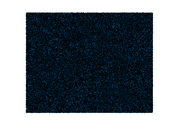### Degree distribution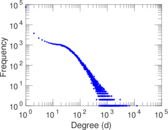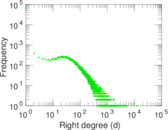### Cumulative degree distribution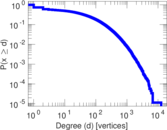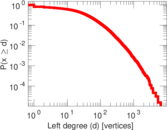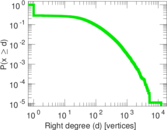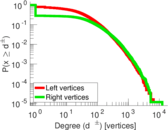### Lorenz curve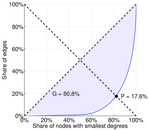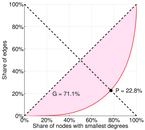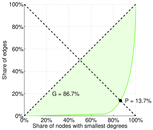### Spectral distribution of the adjacency matrix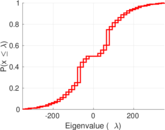### Spectral distribution of the normalized adjacency matrix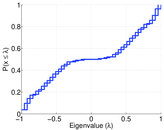### Spectral distribution of the Laplacian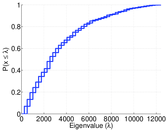### Spectral graph drawing based on the adjacency matrix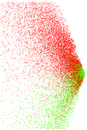### Spectral graph drawing based on the Laplacian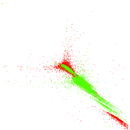### Spectral graph drawing based on the normalized adjacency matrix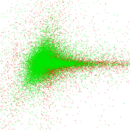### Degree assortativity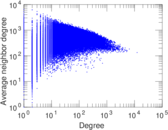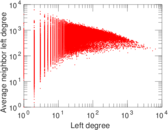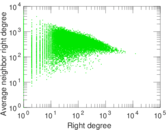### Zipf plot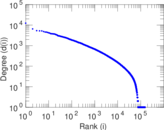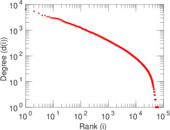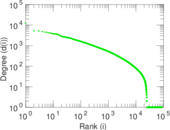### Hop distribution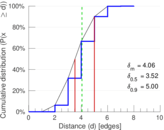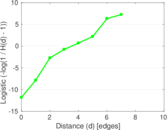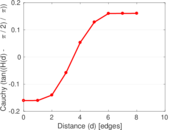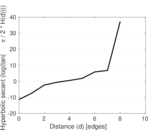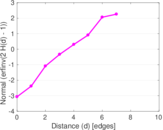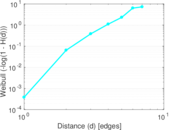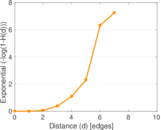### Delaunay graph drawing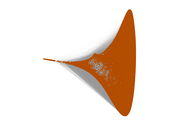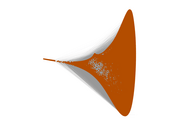### Matrix decompositions plots